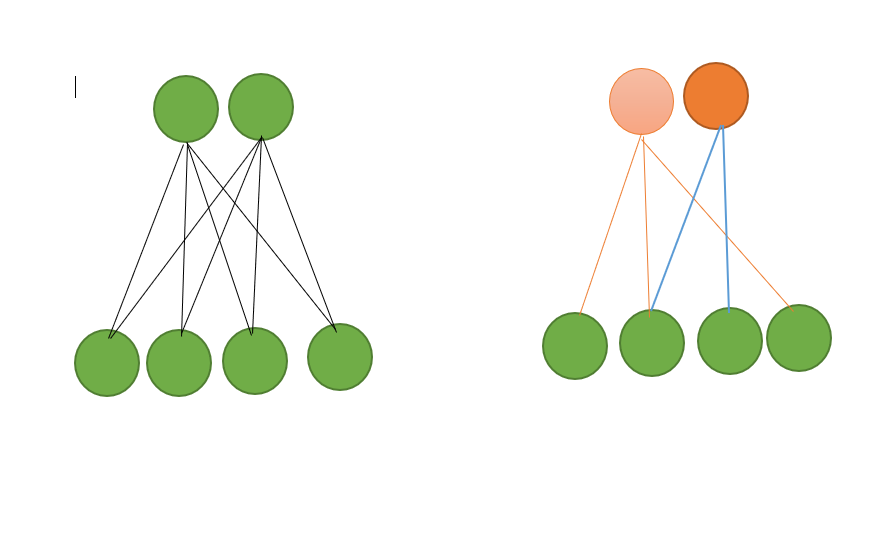# Importance of Convolutional Neural Network | ML

Convolutional Neural Network as the name suggests is a neural network that makes use of convolution operation to classify and predict.

Let’s analyze the use cases and advantages of a convolutional neural network over a simple deep learning network.

Weight sharing:
It makes use of Local Spacial coherence that provides same weights to some of the edges, In this way, this weight sharing minimizes the cost of computing. This is especially useful when GPU is low power or missing.Memory Saving:
The reduced number of parameters helps in memory saving. For e.g. in case of MNIST dataset to recognize digits, if we use a CNN with single hidden layer and 10 nodes, it would require few hundred nodes but if we use a simple deep neural network, it would require around 19000 parameters.

Independent of local variations in Image:
Let’s consider if we are training our fully connected neural network for face recognition with head-shot images of people, Now if we test it on an image which is not a head-shot image but full body image then it may fail to recognize. Since the convolutional neural network makes use of convolution operation, they are independent of local variations in the image.

Equivariance:
Equivariance is the property of CNNs and one that can be seen as a specific type of parameter sharing. Conceptually, a function can be considered equivariance if, upon a change in the input, a similar change is reflected in the output. Mathematically, it can be represented as` f(g(x)) = g(f(x))`. It turns out that convolutions are equivariant to many data transformation operations which helps us to identify, how a particular change in input will affect the output. This helps us to identify any drastic change in the output and retain the reliability of the model.

Independent of Transformations:
CNNs are much more independent to geometrical transformations like Scaling, Rotation etc.

Example of Translation indepence – CNN identifies object correctlyExample of Rotation independence – CNN identifies object correctlyMy Personal Notes arrow_drop_upCheck out this Author's contributed articles.

If you like GeeksforGeeks and would like to contribute, you can also write an article using contribute.geeksforgeeks.org or mail your article to contribute@geeksforgeeks.org. See your article appearing on the GeeksforGeeks main page and help other Geeks.

Please Improve this article if you find anything incorrect by clicking on the "Improve Article" button below.

Article Tags :
Practice Tags :

Be the First to upvote.

Please write to us at contribute@geeksforgeeks.org to report any issue with the above content.